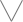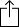C♭
D♭
E♭
F♭
G♭
A♭
B♭
Chord
Scale
Custom
3nps
CAGED
None
Notes
Degrees
Intervals
None

1
2
3
4
5
6
7
1
2
3
4
5
6
7
8
9
10
11
12
13
14
15
16
17
18
19
20
21
22
23
24
E
B
G
D
A
E
B
F
F
G
A
A
B
B
C
C
D
E
E
F
F
G
A
A
B
B
C
C
D
E
E
F
C
D
E
E
F
F
G
A
A
B
B
C
C
D
E
E
F
F
G
A
A
B
B
C
A
B
B
C
C
D
E
E
F
F
G
A
A
B
B
C
C
D
E
E
F
F
G
A
E
F
F
G
A
A
B
B
C
C
D
E
E
F
F
G
A
A
B
B
C
C
D
E
B
C
C
D
E
E
F
F
G
A
A
B
B
C
C
D
E
E
F
F
G
A
A
B
F
G
A
A
B
B
C
C
D
E
E
F
F
G
A
A
B
B
C
C
D
E
E
F
C
D
E
E
F
F
G
A
A
B
B
C
C
D
E
E
F
F
G
A
A
B
B
C
A
A
B
B
C
C
D
E
E
F
F
G
A
A
B
B
C
C
D
E
E
F
F
G

# Cb Minor for 8 String Guitar - 3nps Chart

Cb Minor, sometimes called "Cb Aeolian Mode" is a diatonic scale and is the 6th mode in the set of Classical church modes

C
D
E
F
G
A
B
CW
H
W
W
H
W
W
Play scaleand then